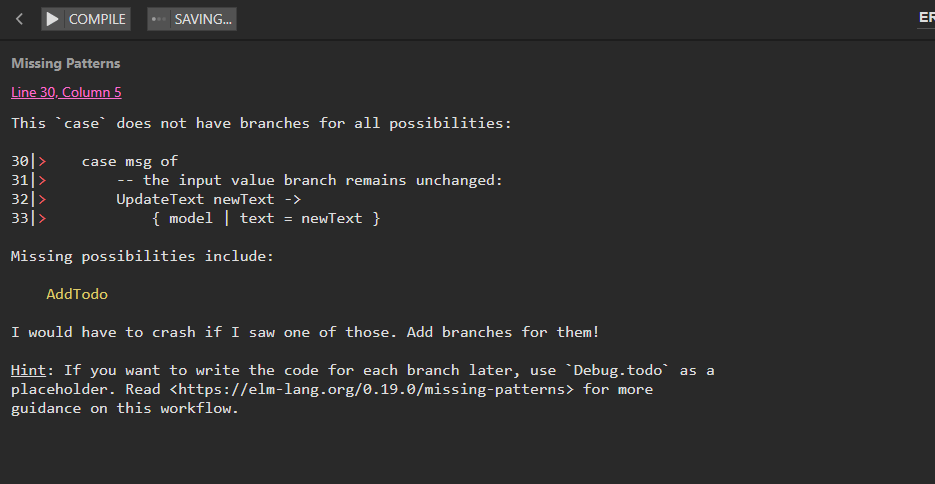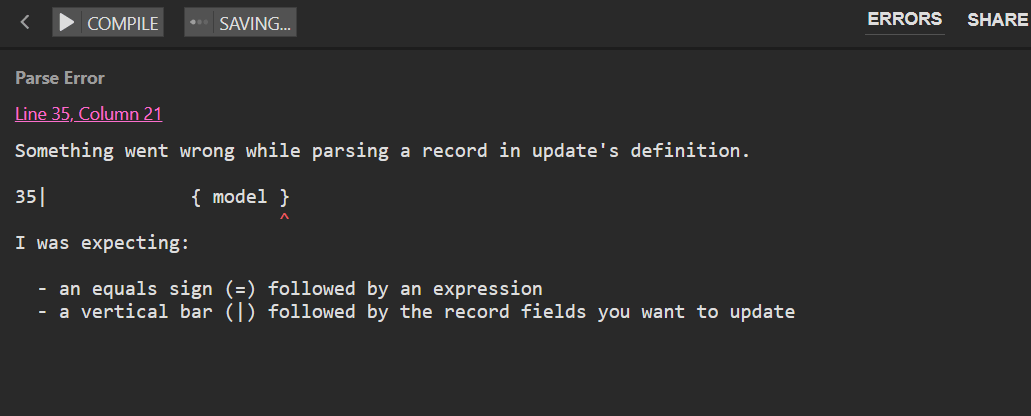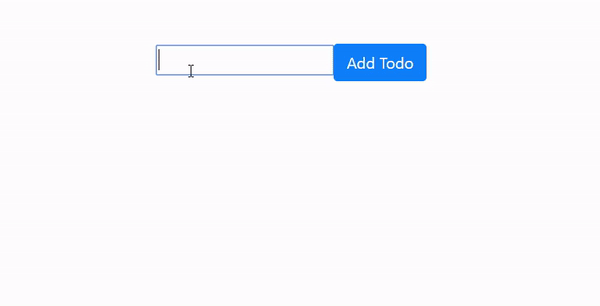In this article, we’ll see how to work with input fields in Elm 0.19.## Starting from the dynamic input example

``````module Main exposing (main)

import Browser exposing (sandbox)
import Html exposing (div, input, text)
import Html.Attributes exposing (class, value)
import Html.Events exposing (onInput)

initialModel =
{ text = "" }
-- (1) we'll add another property on the Record;
-- this new property will store typed-in entries

view model =
div [ class "text-center" ]
[ input [ onInput UpdateText, value model.text ] []
-- (2) we'll be updating the below div
--with the value of new property we added in (1)
, div [] [ text model.text ]
]

type Msg
= UpdateText String

update msg model =
case msg of
-- the input value branch remains unchanged:
UpdateText newText ->
{ model | text = newText }
-- we'll add another pattern to match here;
-- this one updates the model with the new property being added

main =
sandbox
{ init = initialModel
, view = view
, update = update
}
``````

Let’s see this starting app in Ellie:

## Adding a call to the button function inside the view function

Let’s first add the button to our view:

``````view model =
div [ class "text-center" ]
[ input [ onInput UpdateText, value model.text ] []
, button [ onClick AddTodo, class "btn btn-primary" ] [ text "Add Todo" ]
-- (2) we'll be updating the below div
--with the value of new property we added in (1)
, div [] [ text model.text ]
]
``````

This will throw three errors:

``````I cannot find a `button` variable:
...
I cannot find a `onClick` variable:
...
I cannot find a `AddTodo` constructor:
``````

We’ll fix this by:

• importing the `button` function from the `Html` module
• importing the `onClick` event from the `Html.Events` module
• Adding the `AddTodo` constructor to the `Msg` type

Once we fix these errors, we’ll be facing the next set of errors that we’ll need to fix; often, this process is repeated several times before we reach a point where our app simply compiles. This is what I call compiler-driven development.

## Compiler-driven development in Elm 0.19

Let’s fix the three errors, then see what else the compiler will have for us to do.

First, we’ll expose the `button` function in the `Html` import:

``````import Html exposing (div, input, text, button)
``````

Next, we’ll expose the `onClick` event in the `Html.Events` import:

``````import Html.Events exposing (onInput, onClick)
``````

Finally, we’ll add the `AddTodo` type constructor:

``````type Msg
= UpdateText String
``````

Great! We’ve solved our errors, now we’ll hit the compile button in Ellie, and get the `Missing Patterns` error:## Adding a dynamic input with a clickable button in Elm 0.19

Let’s add that missing pattern to the `update` function now:

``````update msg model =
case msg of
-- the input value branch remains unchanged:
UpdateText newText ->
{ model | text = newText }
{ model }
``````

The above code will throw another error; this time, it’s a `Parse Error`:Let’s listen to the suggestion and add the pipe, following it up with the record fields we want to update:

``````update msg model =
case msg of
-- the input value branch remains unchanged:
UpdateText newText ->
{ model | text = newText }
{ model | text = "whatever" }
``````

Now that we’ve pattern matched the AddTodo case, our app compiles and works like this:You can try it yourself in the updated app in Ellie:

Next, rather than just overriding the input with a hardcoded `String` “whatever”, we’ll need to store the input values a user types into the input field.

We’ll store these values whenever a user clicks the “Add Todo” button.

## Storing values in a data structure

To store values, we need some kind of a data structure. We’ll be using a `List`, inside our model’s `Record`:

``````type alias Model =
{ text: String
, todos: List String
}

initialModel =
{ text = ""
, todos = []
}
``````

We had to add the `type alias Model` so that we can define our data structure.

Then in the `initialModel` we give the initial values to both the `text` property in the Record and the `todos` property in the Record. Both values are initially empty: `text` is an empty `String`, and `todos` is an empty `List`.

Next, we’ll update our `todos List` with the hardcoded word “whatever”:

``````update msg model =
case msg of
-- the input value branch remains unchanged:
UpdateText newText ->
{ model | text = newText }
-- the AddTodo value branch should update the todos List
{ model | todos = "whatever" }
``````

This doesn’t really do much for us. Actually, it even throws an error:

Why this error?

Basically, the `update` function’s type annotation looks like this:

``````, update :
Msg
-> { text : String, todos : String }
-> { text : String, todos : String }
``````

However, the `sandbox` needs the `update` argument to be:

``````    , update :
Msg
-> { text : String, todos : List a }
-> { text : String, todos : List a }
``````

Let’s fix this error:

``````update msg model =
case msg of
-- the input value branch remains unchanged:
UpdateText newText ->
{ model | text = newText }
-- the AddTodo value branch should update the todos List
{ model | todos = [ "whatever" ] }
``````

Now our `update` function is in sync with what `sandbox` expects.

Let’s look at the code of our app again:

``````module Main exposing (main)

import Browser exposing (sandbox)
import Html exposing (div, input, text, button)
import Html.Attributes exposing (class, value)
import Html.Events exposing (onInput, onClick)

type alias Model =
{ text: String
, todos: List String
}

initialModel =
{ text = ""
, todos = []
}

view model =
div [ class "text-center" ]
[ input [ onInput UpdateText, value model.text ] []
, button [ onClick AddTodo, class "btn btn-primary" ] [ text "Add Todo" ]
-- (2) we'll be updating the below div
--with the value of new property we added in (1)
, div [] [ text model.text ]
]

type Msg
= UpdateText String

update msg model =
case msg of
-- the input value branch remains unchanged:
UpdateText newText ->
{ model | text = newText }
{ model | todos = [ "whatever" ] }

main =
sandbox
{ init = initialModel
, view = view
, update = update
}

``````

Right now, clicking the Add Todo button doesn’t do anything. Each time we click the Add Todo button, the `todos` variable is set to a `List` of `Strings`, which holds a single, hardcoded `String`: “whatever”.

Next, we’ll see how to update the `todos` List, so that we can actually show it.

## Showing the List of todos

Let’s try to show the List of todos.

### A naive approach

Let’s try to update the `view` function like this:

``````view model =
div [ class "text-center" ]
[ input [ onInput UpdateText, value model.text ] []
, button [ onClick AddTodo, class "btn btn-primary" ] [ text "Add Todo" ]
, div [] [ List.map listToString model.todos ]
]
``````

What we’re doing above is, we’re running the `List.map` function and giving it two parameters:

• the mapping function (the “transformer” function), `listToString`
• the `model.todos` List that we’ll be mapping over; this `model.todos` List is the source data structure for our `List.map` function to work on

Next, we’ll need to define the mapping function, `listToString`:

``````listToString todo =
div [] [ text todo ]
``````

The `listToString` function takes a `todo` and returns a `div` with the `todo` value as this div’s text node.

Why doesn’t this code work:

``````listToString todo =
div [] [ text todo ]

view model =
div [ class "text-center" ]
[ input [ onInput UpdateText, value model.text ] []
, button [ onClick AddTodo, class "btn btn-primary" ] [ text "Add Todo" ]
, div [] [ List.map listToString model.todos ]
]
``````

The error we get is this:

``````Type Mismatch
Line 27, Column 18
The 2nd argument to `div` is not what I expect:

27|         , div [] [ List.map listToString model.todos ]
^^^^^^^^^^^^^^^^^^^^^^^^^^^^^^^^^^^^^
This argument is a list of type:

List (List (Html.Html msg))

But `div` needs the 2nd argument to be:

List (Html.Html msg)
``````

As we can see, we are nesting a List inside a List, while the compiler expects the div’s second argument to be just a regular List, not a nested one.

Here’s the fix:

``````, div [] ( List.map listToString model.todos )
``````

Note: We’re using `(` and `)` to group code in Elm 0.19.

Now our app works again, but we’re not storing the todo we mapped over with `List.map`.

The reason is the `update` function’s `AddTodo` branch: it currently has a hard-coded `String` in a `List`:

``````AddTodo ->
{ model | todos = [ "whatever" ] }
``````

Let’s update it like this:

``````AddTodo ->
{ model | todos = model.todos }
``````

This update doesn’t break the app; it still compiles.

However, it doesn’t print anything to the screen now:

Why is that?

It’s becuase we’re simply assigning the starting value of `model.todos` to our newly updated `todos` variable in this line of code:

``````AddTodo ->
{ model | todos = model.todos }
``````

In the code above, we’re effectively saying:

1. Take the empty List that’s stored in model.todos: `model.todos`, and
2. assign that value to the model’s `todos` property: `model | todos =`

Basically, we’re just re-assigning the existing value to itself.

``````AddTodo ->
{ model | todos = model.todos ++ [ "whatever" ] }
``````

What we’re doing now is this: whenever a user clicks a button, we add a `String` in a `List` to `model.todos`.

Now, for the Add Todo button to work, the input doesn’t even have to be typed into. We can just click the button, and every time we do, we’ll get another word “whatever” printed to the bottom.

Now all that’s left to do is add the value of the input in place of the hardcoded “whatever” String.

## Update model.todos with the typed-in String

This update is minimal:

``````AddTodo ->
{ model | todos = model.todos ++ [ model.text ] }
``````

The app now works, but we need to clear the input on each button click.

## Making the input reset whenever the AddTodo message is sent

This is a tiny update too:

``````update msg model =
case msg of
UpdateText newText ->
{ model | text = newText }
-- We append the model.text value to the end of our list of todo strings.